Let $\theta$ denote the angular displacement of a simple pendulum oscillating in a vertical plane. If the mass of bob is m. The tension in the string is mg cos $\theta$

1.  always

2.  never

3.  at extreme

4.  at mean position

Concept Questions :-

Non-Uniform vertical circular motion
High Yielding Test Series + Question Bank - NEET 2020

Difficulty Level:

In a uniform circular motion, which of the following quantity is not constant

(1) Angular momentum

(2) Speed

(3) Kinetic energy

(4) Momentum

Concept Questions :-

Uniform circular motion
High Yielding Test Series + Question Bank - NEET 2020

Difficulty Level:

The graph for stopping time (t) versus mass (m) is shown in the figure. Then which one of the pair is given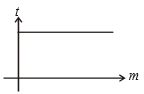1.  Momentum, force

2.  K.E., force

3.  Velocity, force

4.  None of these

Concept Questions :-

Application of laws
High Yielding Test Series + Question Bank - NEET 2020

Difficulty Level:

A metal ring of mass m and radius R is placed on smooth horizontal table and is set rotating about its own axis in such a way that each part of the ring moves with a speed v. Tension in the ring is:

(1) $\frac{m{v}^{2}}{R}$

(2) $\frac{m{v}^{2}}{2R}$

(3) $\frac{m{v}^{2}}{2\mathrm{\pi }R}$

(4) None of these

Concept Questions :-

Uniform circular motion
High Yielding Test Series + Question Bank - NEET 2020

Difficulty Level:

Tension on the string at point P is T. The graph for tension (T) versus x is shown in the figure. Then the string is: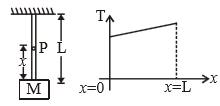1.  massless

2.  massfull

3.  tension on every point on the string is same when the string is having finite mass 4

4.  None of these

Concept Questions :-

Application of laws
High Yielding Test Series + Question Bank - NEET 2020

Difficulty Level:

While walking on ice one should take small steps to avoid slipping. This is because smaller steps ensure

(1) Larger friction

(2) Smaller friction

(3) Larger normal force

(4) Smaller normal force

Concept Questions :-

Newton laws
High Yielding Test Series + Question Bank - NEET 2020

Difficulty Level:

Forces of 1 N and 2 N act along with the lines x = 0 and y = 0. The equation of the line along which the resultant lies is given by

1.  y - 2x = 0

2.  2y - x = 0

3.  y + x = 0

4.  y - x = 0

Concept Questions :-

Newton laws
High Yielding Test Series + Question Bank - NEET 2020

Difficulty Level:

A block is kept on a rough inclined plane with angle of inclination $\theta$. The graph of net reaction (R) versus $\theta$ is:

1.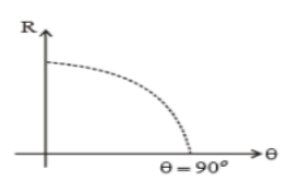2.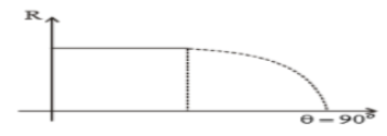3.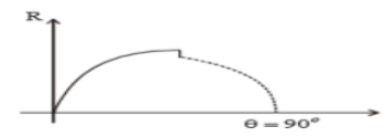(4) None of these

Concept Questions :-

Application of laws
High Yielding Test Series + Question Bank - NEET 2020

Difficulty Level:

A block is placed on a rough horizontal plane. A time dependent horizontal force F=kt acts on the block. The acceleration time graph of the block is :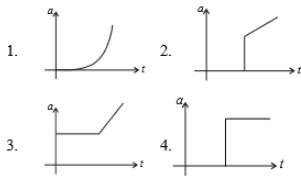Concept Questions :-

Application of laws
High Yielding Test Series + Question Bank - NEET 2020

Difficulty Level:

A particle is moving in a vertical circle. The tension in the string when passing through two positions at anele of 30$°$ and 60$°$ from vertical (the lowest position) are respectively, then:

1.

2.

3.

4.  Tension in the string always remains the same.

Concept Questions :-

Non-Uniform vertical circular motion